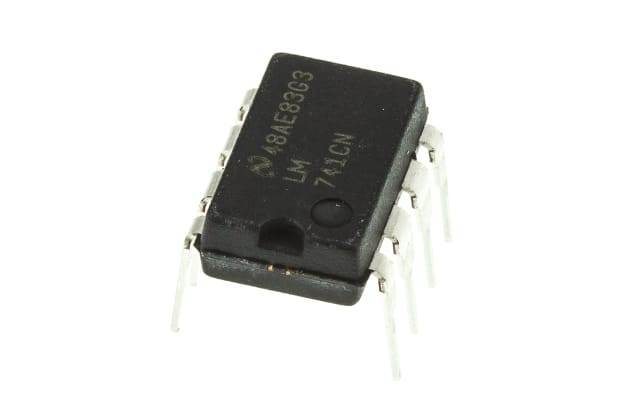Recently searched

# What are Op Amps?

Discover exactly how operational amplifiers, or Op Amps, work and how they can be used.Operational amplifiers, otherwise known as op amps, are electrical components designed to carry out a variety of different tasks. With two inputs and a single output, these semiconductor devices can be combined with other op amps, resistors and capacitors depending on the desired function of the circuit.

Used in DC power circuits, the main way op amps are used is to amplify the difference between the signal of its inverting and non-inverting inputs. Plus, op amps are available in inverting and non-inverting forms. These characteristics make op amps one of the most useful and widely-used components in electronic circuits.

In this guide, we will explain what an op amp is, the different types, applications and how they work.

## How Do Op Amps Work?The characteristics of op amps are:

• Gain that’s mostly infinite
• High input impedance (which is mostly infinite)
• Low output impedance (usually around 0)

Under differential input, the output of op amps is the proportional difference between its two inputs. When the same input is applied, the output should not change.

## Types of Op Amps

Op amps can be categorised based on various factors, such as:

Power supply configuration:

Input and output configuration:

• Inverting op amps
• Non-inverting op amps

Internal architecture:

• Bipolar Junction Transistor (BJT) based op amps
• Field-Effect Transistor (FET) based op amps
• Complementary Metal-Oxide-Semiconductor (CMOS) based op amps

Op amps can come in different variations, depending on the task required. The first of these amplifying circuits is a dual power supply op amp. This amplifying circuit has input from two different power supplies and amplifies the difference between these power supplies.

The dual supply amplifier has an AC signal input and positive voltage as one power supply and a negative voltage as another power supply. These power supplies create the range in which the output amplified current can alternate. Because of the second negative power supply, a full waveform can be created at the amplifier output.

This is different to a single power supply op amp. In this circuit, there is only one positive supply, with a ground acting as a negative power supply. Like the dual supply operational amplifier, the circuit has an alternating input. However, unlike the dual supply amplifier, the output signal is not a full waveform.

This is because the negative charge inputted into the amplifier circuit is ground, meaning that the current can only alternate to a voltage as low as zero volts. To summarise, the range of the outputting alternating signal is reduced in a single power op amp, due to its singular power supply.

### Non-Inverting Amplifier

Among the other types of operational amplifiers, there is also an op amp type called a non-inverting amplifier. These op amps are given their name due to their waveform characteristics.

The waveform on output will have the same signal highs and lows as the input signal; it will also mirror the waveform at the same speed or frequency. The term for this mirroring of input and output signals is also known as the input and output signals being in phase.

As well as the in-phase characteristics, the non-inverting operational amplifier also uses resisters as an input method in order to create an amplified output. The amplified output from this method of the circuit is referred to as an amplifier gain.

A non-inverting amplifier gain can be calculated using the equation gain= 1+(r1/r2). The variance of the resistors is what creates the amplification in a non-inverting amplifier, as there is no current running through the input.

Therefore, the voltage in and voltage out remains the same, and the gain amount is dependent on the variance in the resistors, though the gain from this amplifier will never be less than 1, due to its voltage level.

### Inverting Amplifier

In contrast to the non-inverting operational amplifier, the inverting amplifier does have differences between its input and output signals. In the inverting op amp, the output and input signals are out of phase with each other.

If the input in an inverting amplifier is positive, its output will be negative. Likewise, if the input signal is negative, then its output will be positive. The waveform of the inverting amplifier is also visually out of phase with the inputs, as its waves peak at higher positive voltages and drop to lower negative voltages.

Plus, due to its differing waveforms, or out-of-phase characteristics, the inverted operational amplifier gain is calculated using a different equation. Like the non-inverting amplifying circuit, the Inverting op amp uses resistors to create an output. However, this output is calculated from the resistor feedback and input resistor, with no additional input or +1.

To summarise the amplifier gain equals the resistor input vs the resistor frequency, with minus shown in the equation to represent the phase-out. The equation for an inverting op amp is written as AV=-R1/R2.

## Op Amps ApplicationsDue to their function and versatility, operational amplifiers can be found in virtually every electronic device. They are also used in public, domestic, commercial, and industrial sectors.

Examples of where op amps have been used include alarms, light sensors, radios, and sending a chain of signals which can also be used in control applications to control machinery. Op amps are also great for audio sensing technology. Non-inverting op amps are present in mixing desks, and can also be found in computers and processing circuits, display screens and communication tools.

Finally, non-inverting op amps can be used as phase shifters, whilst op amps in general can be used as inverters, comparators and as part of inversion circuits. Operational amplifier circuits are an integral function part of most electronics used in the modern day. Moreover, because operational amplifier circuits are designed to amplify the weakest signals, it is not uncommon to find multiple op amps in one electronic device, where a greater output is needed.